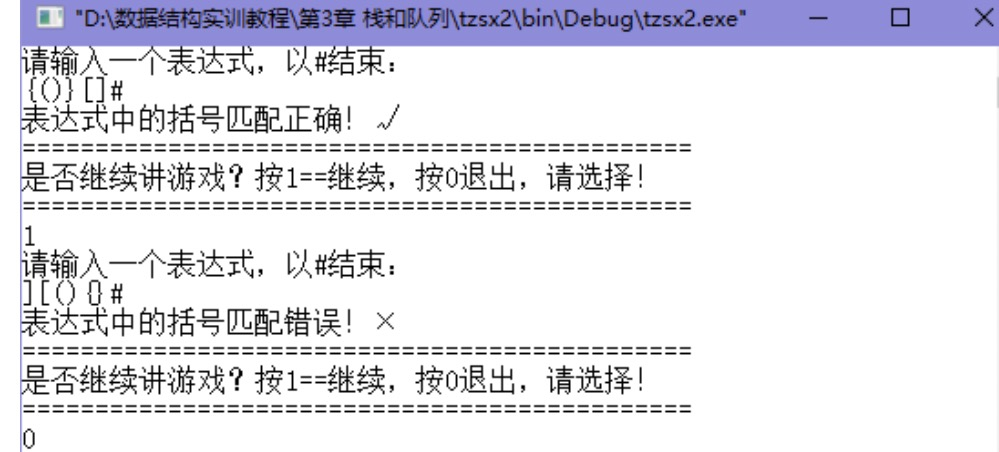2021-06-23 00:47

# c语言用栈实现括号匹配• 写回答
• 关注问题
• 收藏
• 邀请回答

#### 1条回答默认 最新

•hubei_dxx 2021-06-24 09:21
已采纳
``````#include <stdio.h>
#include <stdlib.h>
#include <string.h>

#define CSTACK_SIZE 100
struct CStack
{
char e[CSTACK_SIZE];
int  top;
};

struct CStack* CreateStack()
{
struct CStack *pStack = (struct CStack*)malloc(sizeof(struct CStack));
pStack->top = -1;
return pStack;
}

void DestroyStack(struct CStack* s)
{
free(s);
}
void PushStack(struct CStack* s, char c)
{
if (s->top >= CSTACK_SIZE)
{
printf("stack is full\n");
return;
}
s->top++;
s->e[s->top] = c;
}

char PopStack(struct CStack* s)
{
char c = '\0';
if (s->top < 0)
{
printf("stack is empty\n");
return c;
}

c = s->e[s->top];
s->top--;
return c;
}

int IsEmpty(struct CStack* s)
{
if (s->top < 0)
{
return 1;
}
else
{
return 0;
}
}
char GetTop(struct CStack* s)
{
if(IsEmpty(s))
{
return '\0';
}
return s->e[s->top];
}
int match(char a,char b){
if(a+1==b||a+2==b)//成对的左右括号的ASCII码相差1或者2
return 1;
return 0;
}
int check(char str[])
{
int  i, len = 0;
char c;
struct CStack* s;
len = strlen(str);
s = CreateStack();
for (i = 0; i < len; i++)
{
switch(str[i])
{
case '{':
case '[':
case '(':
PushStack(s, str[i]);
break;
case '}':
case ']':
case ')':
if (IsEmpty(s))
{
DestroyStack(s);
return 0;
}
else
{
c = GetTop(s);
if (match(c, str[i]))
{
PopStack(s);
}
else
{
DestroyStack(s);
return 0;
}
}
}
}

if (IsEmpty(s))
{
DestroyStack(s);
return 1;
}
else
{
DestroyStack(s);
return 0;
}
}

int main(int argc, char* argv[])
{

char str = {0};
char c='1';
const char* msg =
"==========================================\n"
"是否继续游戏？按1==继续，按0退出，请选择!\n"
"==========================================\n";

do
{
gets(str);
switch(strlen(str))
{
case 1:
c = str;
break;
default:
if(check(str) == 1)
{
printf("匹配正确！\n%s", msg);
}
else
{
printf("匹配错误！\n%s", msg);
}
}
} while (c!='0');

return 0;
}``````
已采纳该答案
1 打赏 评论